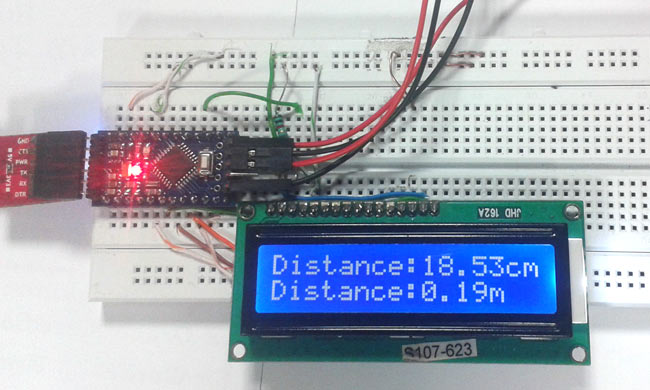# Arduino Based Distance Measurement using Ultrasonic Sensor

Ultrasonic sensors are great tools to measure distance without actual contact and used at several places like water level measurement, distance measurement etc. This is an efficient way to measure small distances precisely. In this project we have used an Ultrasonic Sensor to determine the distance of an obstacle from the sensor. Basic principal of ultrasonic distance measurement is based on ECHO. When sound waves are transmitted in environment then waves are return back to origin as ECHO after striking on the obstacle. So we only need to calculate the travelling time of both sounds means outgoing time and returning time to origin after striking on the obstacle. As speed of the sound is known to us, after some calculation we can calculate the distance.### Components Used

1. Arduino Pro Mini
2. Ultrasonic sensor Module
3. 16×2 LCD
4. Scale
6. 9 volt battery
7. Connecting wires

### Ultrasonic Sensor Module

Ultrasonic sensor HC-SR04 is used here to measure distance in range of 2cm-400cm with accuracy of 3mm. The sensor module consists of ultrasonic transmitter, receiver and the control circuit. The working principle of ultrasonic sensor is as follows:

1. High level signal is sent for 10us using Trigger.
2. The module sends eight 40 KHz signals automatically, and then detects whether pulse is received or not.
3. If the signal is received, then it is through high level. The time of high duration is the time gap between sending and receiving the signal.

Distance= (Time x Speed of Sound in Air (340 m/s))/2Timing Diagram

The module works on the natural phenomenon of ECHO of sound. A pulse is sent for about 10us to trigger the module. After which the module automatically sends 8 cycles of 40 KHz ultrasound signal and checks its echo. The signal after striking with an obstacle returns back and is captured by the receiver. Thus the distance of the obstacle from the sensor is simply calculated by the formula given as

Distance= (time x speed)/2.

Here we have divided the product of speed and time by 2 because the time is the total time it took to reach the obstacle and return back. Thus the time to reach obstacle is just half the total time taken.

Read MoreRead More:   Arduino Based Distance Measurement using Ultrasonic Sensor

#### This Post / Project can also be found using search terms:

• arduino and ultrasonic sensor based distance measurnment system